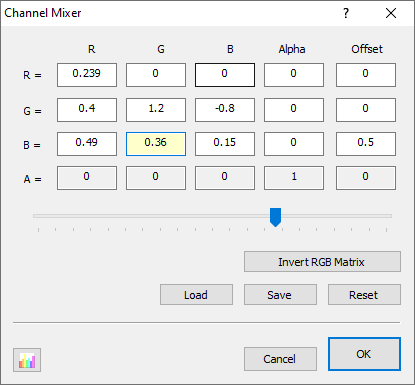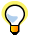...Channel Mixer

Channel Mixer adjustment does exactly does what its name implies; it mixes color channels. You can use it to repair bad channels, create special effects, tint images, simulate color conversions and even produce grayscale images from color images based on a custom algorithm. You can also use it to swap or duplicate channels. The user interface is laid out like a 5-by-4 matrix showing how the input channels are summed to creates the output. Each takes a multiplier with denotes that contribution of that channel to the final output, with 1 representing 100% contribution. When you select any specific value, a slider is shown at the bottom via which you may easily manipulate that value.The Math

As mentioned before, the filter is controlled through a 5-by-4 matrix. This is applied as follows:

 red = a[ 0] * srcR + a[ 1] * srcG + a[ 2] * srcB + a[ 3] * srcA + a[ 4] green = a[ 5] * srcR + a[ 6] * srcG + a[ 7] * srcB + a[ 8] * srcA + a[ 9] blue = a * srcR + a * srcG + a * srcB + a * srcA + a alpha = a * srcR + a * srcG + a * srcB + a * srcA + a

As you can see, the values in the first row determine the resulting red value, the second row the green value, the third row the blue value, and the fourth row the alpha value. You can also see that the values in the first four columns are multiplied with the source red, green, blue, and alpha values, respectively; while the fifth column value (offset) is added as is.Note that the source and result values for each channel range between 0 and 255. So even though the resulting value of red, green, or blue can be greater than 255 or less than 0, the values are constrained to this range.